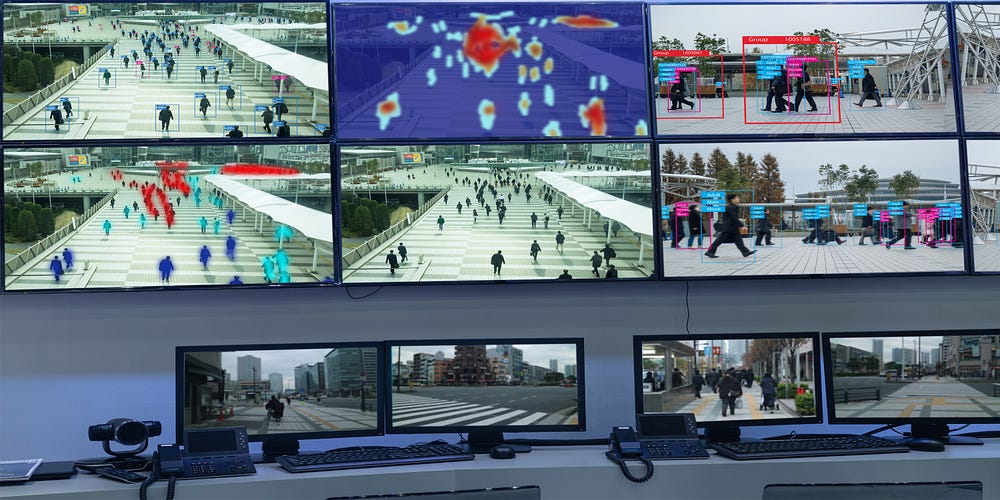# Brief Overview on object detection Algorithms.

Source: Deep Learning on Medium

## Understanding Object detection frameworks and discussing the evolution of the same.

At Fractal Analytics, We worked on several object detection problems. We have used a variety of frameworks available in the open-source community, modified it according to our use case and trained on our own data to build applications for various clients across different domains. We worked on a variety of images which include natural landscape images, traffic congestion images, satellite image, Retail store images and Aisle analytics etc in the last one year span. We partly used our object detection framework in our video and images classification problems too, this partly improved our results with the additional bottleneck of annotating data-sets.

While there is a ton of material available online, knowing where to get started is still difficult. We want to document everything we have ever read and found useful. We are writing this with a hope that it will help someone.

To begin with, object detection is mostly an unsolved problem with a lot of improvements going to come over the next few years. While Image classification accuracies are touching an top 5 error rate of 2.25% on ImageNet challenge and the community itself closed the competition and declared that we have achieved greater than human level accuracy, Object detection is in a very naive stage, with state of the art object detection algorithms achieving only 40.8 mAP (100 is the max) on COCO datasets, we are still a long way to achieve this. Knowing exactly where these algorithms fail and carefully curating the datasets for your particular use case should give good results even in the object detection challenges. This blog post is about how the deep learning research community has come to a stage where it is now. We will discuss the key research papers and techniques which helped improve the state-of-the-art results at the time and how they influenced researchers in exploring new frontiers. All in all we believe reading this blog post should give a good overview of what is happening inside the research community within the object detection algorithms phase.

We will be discussing the following papers,

### Paper Discussions

Traditionally Object detection is done using template matching. Objects used to be cropped and using descriptors like HOG and SIFT, features used to be generated. Then a window used to slide on the image and compare each location with these database of object feature vectors. Some people have gone forward and trained SVM classifiers and replaced these databases. Since objects will be of different sizes, people used different window sizes and image sizes (Image Pyramids). Such complex pipelines used to give decent results but had so many draw backs. It is computationally very time consuming. Hand engineering features using algorithms like HOG and SIFT are not that accurate. So Image processing researchers had a very hard time dealing with this.

With the advent of deep learning and its staggering results in Image classification challenge in 2012 have raised hopes again and different researchers took different paths to solve object detection problem using deep learning. In the line of that, The first paper ever published (At least the one I read and found useful) on object detection using deep learning is R-CNN ( Region proposals with CNN)

#### R-CNN

• This is the first paper to show that CNN can lead to dramatically higher Object detection performance. It achieved 58.5 mAP on VOC 2007 dataset.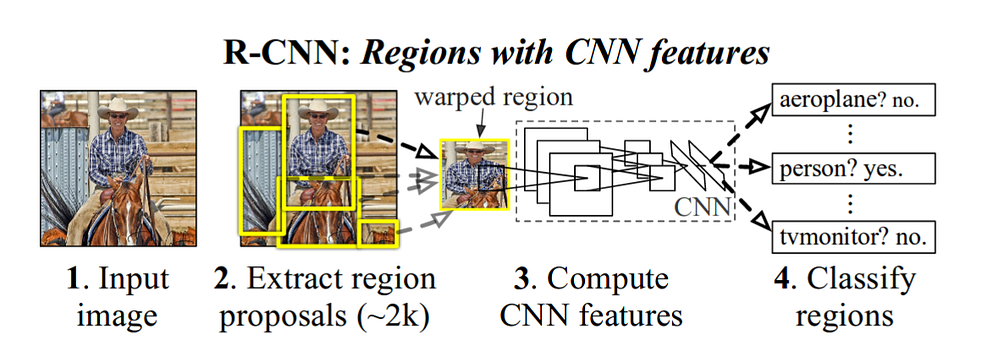R-CNN Architecture.

If you want to build R-CNN in your lab, these are following steps you need to follow

• Take a standard network like VGG or ResNet pre-trained on Image-net. This will act like a feature extractor from the image. We need to remove the class specific classification layer and use the bottleneck layer to extract features from the image. In R-CNN they have used VGG network and we get a 4096-dim vector for each image proposal.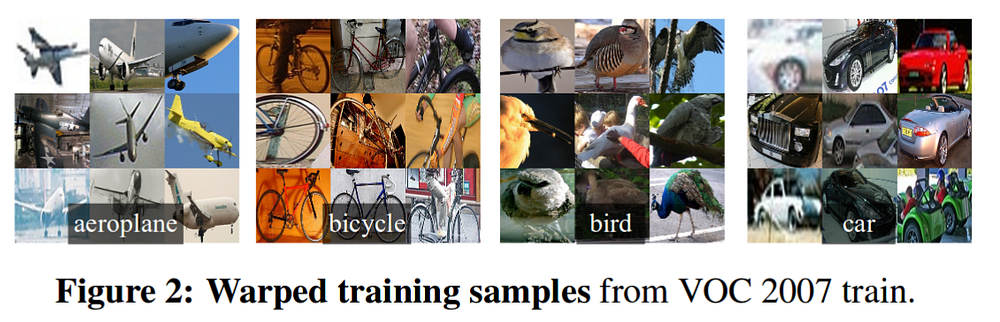RCNN objects
• To make sure that the features are extracted accurately, they train the network using these warped images. While training VOC dataset, they place 20+1 (n_class + background) layer at the end and train the network. Each batch size contains 32 positive windows and 96 background windows. A Region proposal is said to be positive if it has an IoU ≥ 0.5 with the ground truth box. Usually obtain these 128 (32+96) proposals from 2 images (batch size). Since there will be ~2k proposals from each image, we sample the positive images and negative images(background) images separately. We use selective search to generate these proposals.
• After Fine-tuning the network, we will send the proposals through the network and obtain a 4096 dim vector. The authors of this paper performed a grid search on IOU to select the positive samples, IOU of 0.3 worked well for them.
• For each object class, train a SVM (one versus other) classifier. You can use hard negative mining to improve the classification accuracy.
• We also train a bounding box regressors to improve upon the localization errors. This is applied on the proposal once the class specific SVM classifier classify the object.

#### Test Time

• At test time, R-CNN generates around 2000 category-independent region proposal from the input image, extracts a fixed-length feature vector for each proposal using a CNN (VGG Net), and then classifies each region with category specific linear SVM’s. This gives class specific objectness score for each proposal, which are then sent a non-maxim suppression algorithm.
• Test time inference takes 13 sec per image on GPU and 53s/image on CPU.

The major problem with this approach is

• Sending ~2000 proposals to the Neural network, thus bringing test time to 13 sec on GPU.
• Complex pipeline for training and inference. No end to end training pipeline. Neural network is trained separately, SVM classifiers are trained individually.
• These are slightly overcome in SPP net.

### SPP-Net

The major problems with R-CNN is computing feature vector for ~2000 proposals individually by passing each of them separately to the network. Also, when passing the image to the VGG-net, we need a fixed size image shape(224 * 224) and to achieve that we either warp or crop the image, SPP removes this constraint using a spatial pyramid pooling layer. We will look into these two things in this section.

#### spatial pyramid pooling layer

This is not specific to object detection but helps in general to train any neural network. Every CNN takes fixed size image because the end FC layers expect the same size input. this can be understood looking at the following example

Say for an input size of 224 image size, conv5 (last conv layer) has 13*13 feature map and for another input size of 180, the size of conv5 layer is now 10*10. If you apply simple pooling of 2*2, the output size will be 7*7 and 5*5 for input size of 224 and 180 respectively. transforming this will convert the network will give 49*256 (256 feature maps) and 25*256 size layer in the end. The weight matrix attached to the FC layer cannot work on this as it expects a fixed feature vector. So SPP layer comes into our rescue, we use l l-level pyramid pooling layer.

suppose the size of the feature map is a*a and pyramid level of n*n. The stride is floor(a/n) and window size is ceil(a/n). for a pyramid level of 3, we have bins of 3×3, 2×2, 1×1. Below diagram shows further computations.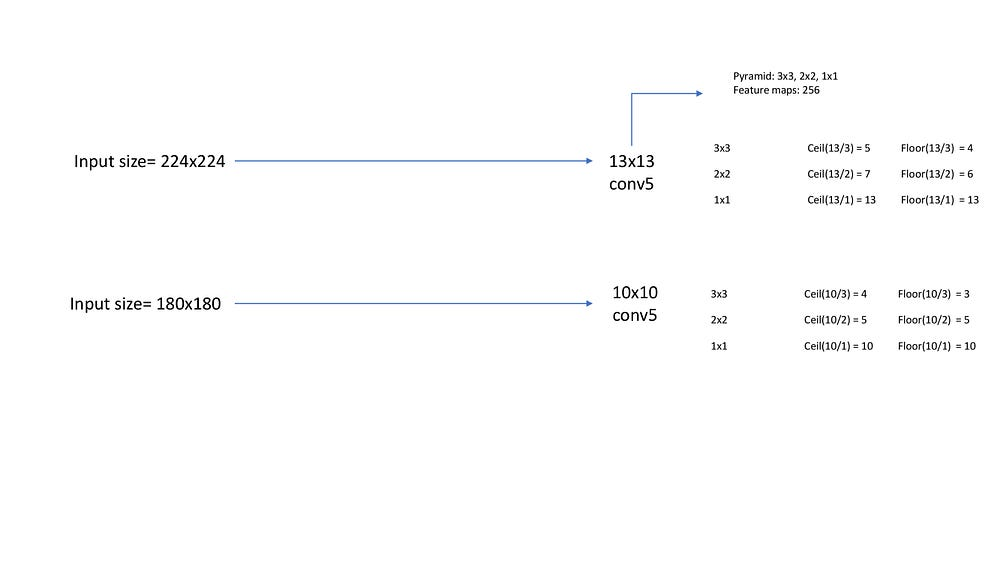Spatial Pyramid pooling

For computations purpose, they have changed the size only after each epoch. Though SPP is good, these are now old fashioned now with global average pooling layers taking their position.

#### SPP-Net For Object detection

As already said, Feature extraction is the major timing bottleneck in testing. So In SPP-Net they apply the CNN network on the entire image and extract the feature map (conv5), for an input size of 416×416 (They actually used 5 scales while training the network) the feature map size at conv5 is 26*26 (subsampled by 16).

Next, we will generate ~2000 proposals using selective search. For each proposal we need to extract a fixed length feature vector from the feature map. This is applied in the following way.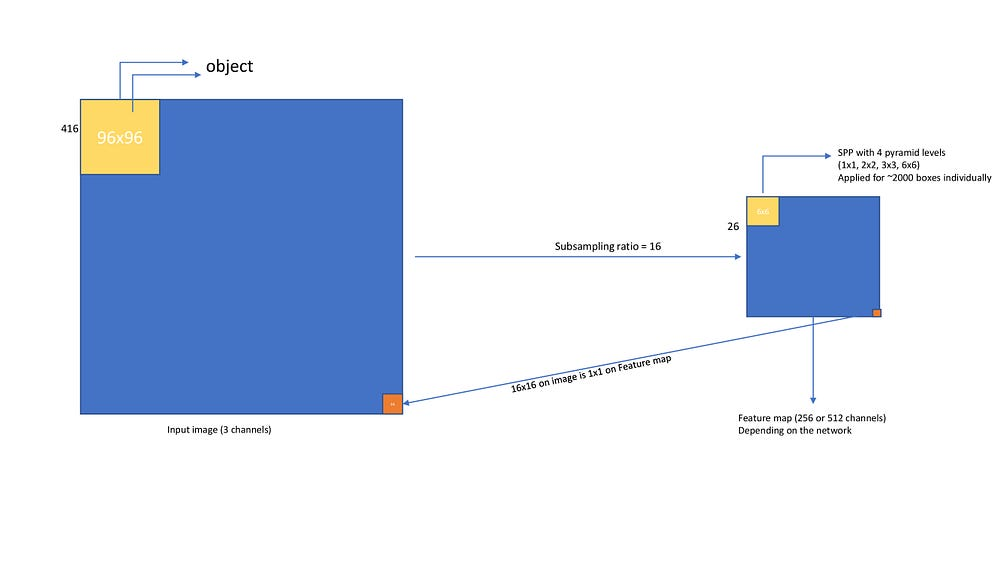Spatial pyramid pooling network

Now, after every proposal gets a fixed length feature vector, we can train SVM classifiers using the same techniques as done in R-CNN.

#### Results

• Using ZF-Net with SPP they have achieved 59.2% mAP on VOC dataset. using multi-scale it achieved 63.1% mAP.
• Inference on SPP network at single scale takes 0.053 sec compared to 14.37s by R-CNN. At 5 scale, it takes 0.29 sec which is still 49x faster.

### Fast R-CNN

The legacy continues and this paper has set pretty good standards for most of the upcoming papers.

#### Major differences brought by Fast R-CNN to SPP-Net are

1. Removed SVM classifiers and joined a regression and classification layer to the net. Thus making it a single stage training and inference network
2. Remove SPP pooling layer and joined it with ROI pooling layer.
3. Use VGGNet as the backend rather than ZFNet used by SPP-Net.
4. Build new loss functions which are less sensitive to outliers.

#### Fast R-CNN network works in the following way

• The network processes the whole image to produce a convolutional feature map. Then for each object proposal ROI pooling layer extracts fixed-length feature vector, which is finally passed to subsequent FC layers.
• The FC layers branch into two sibling output layers, one that estimates softmax probability over K+1 object classes, and another layer producing the refined bounding box positions.
• 2000 proposals of particular image are not passed through the network as in R-CNN, Instead, The image is passed only once and the computed features are shared across ~2000 proposals like the same way it is done in SPP Net .
• Also, the ROI pooling layer does max pooling in each sub-window of approximate size h/H X w/W. H and W are hyper-parameters. It is a special case of SPP layer with one pyramid level.
• The two sibling output layers’ outputs are used to calculate a multi-task loss on each labeled ROI to jointly train for classification and bounding-box regression.
• They have used L1 loss for bounding box regression as opposed to L2 loss in R-CNN and SPP-Net which is more sensitive to outliers.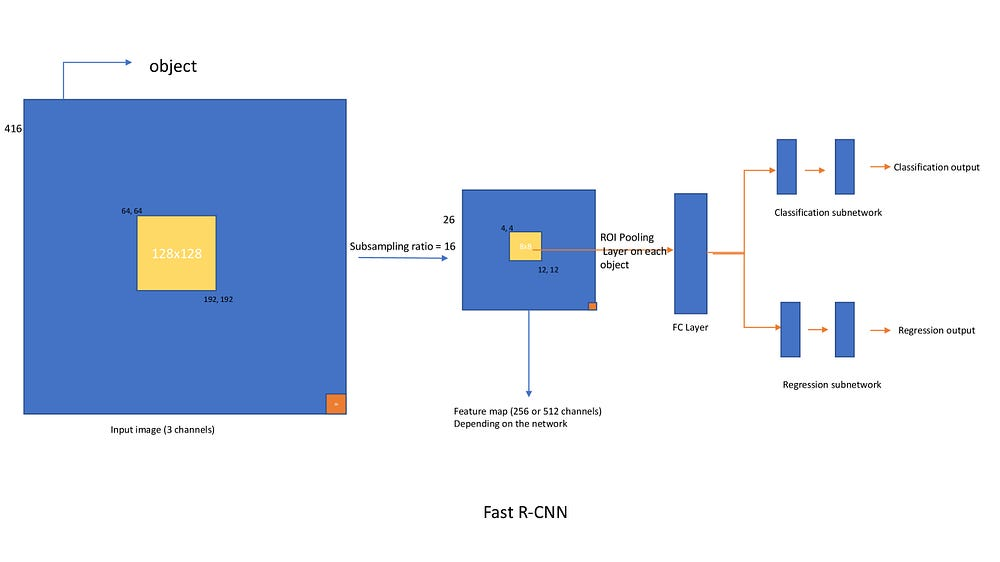Fast R-CNN Network

#### RoI Pooling

Aka Region of Interest pooling, It is important to understand as it is used in both Faster R-CNN and Mask R-CNN.

problem: since objects are of different sizes, the pooling layer needs to be applied on different sizes of feature maps. Suppose in the above diagram the object location [64, 64, 192, 192] on image is [4, 4, 12, 12] on feature map. object location [ 128, 128, 384, 400] on image is [8, 8, 24, 25] on feature map. Now both pooling layer should be applied on both [4, 4, 12, 12] and [4, 4, 16, 18] and extract a fixed length feature vector.

Here is where RoI pooling layer comes to your rescue. Below is the diagram on how it works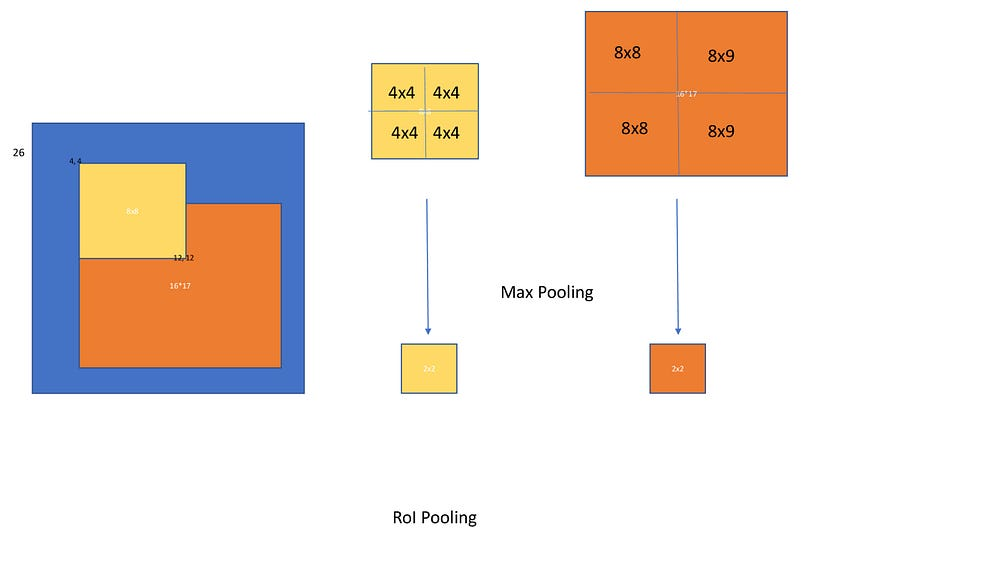ROI Pooling

#### Loss functions

The Fast R-CNN network has two sibling networks as discussed above, the classification layer outputs discrete probability scores and the regression layer outputs offsets of x, y, w, h values of the object. For classification we can use cross entropy loss. for regression they use smooth L1 loss.

#### Training

While training Fast R-CNN uses batch size of 2. Since for a batch size of 2 we have ~2000 x 2 = 4000 boxes and majority of them won’t contain the object, there will be extreme class imbalance while training this network. For this sake, we randomly sample 64 +ve boxes and 64 -ve boxes from the ~4000 boxes and compute losses only for these boxes. Incase if we have less +ve boxes, we will pad with negative boxes. In total we will have 128 boxes for each iteration. The same approach is used in Faster R-CNN also.

#### Encoder

A Proposal is said to be foreground if it has iou > 0.5 with any of the bounding box, we will assign class to the proposal accordingly. proposals which has [0.1, 0.5) are termed to be negative. Remaining all proposals are ignored

#### Results

• Training is 9x faster than R-CNN. While inference Fast R-CNN is 213x faster than R-CNN. Similarly using VGG16, Fast R-CNN is 3x faster while training and 10x faster while inference compared to SPP Net.
• mAP on VOC-2007–12 dataset achieves 71.8%. On COCO Pascal-style mAP is 35.9. The new coco-style mAP, which averages over IoU thresholds, is 19.7% on coco dataset

### Faster R-CNN

The legacy has reached a new heights here. It is still the best choice for most of the researchers and has achieved incredible results. This has surpassed all the previous results in-terms of both accuracy and speed. Faster R-CNN also has come up with new techniques which have become gold standards for all upcoming frameworks. Lets have deep look into these methods.

#### Changes to the Fast R-CNN

• Removed Selective search and added a Deep Neural network for generating proposals (RPN network)
• Introduced anchor boxes

These are the two major changes brought by Faster R-CNN. Anchor boxes became very common from here for all the frameworks. RPN network can work as single object detector or generate proposals for Fast R-CNN network. One thing is for sure, we have removed the traditional computer vision techniques completely and made a full fledged Deep neural network which gets trained end to end.

#### How RPN Works?

• Take an input image of 800×800 and send it to the network. For a VGG Network, after subsampling ratio of 16, the output will be [512, 50, 50]. An RPN network is applied on this feature map, which generates (50*50*9) boxes regression and classification scores. So regression output is 50*50*9*4 (x,y, w, h) and classification output is 50*50*9*2 (object present or not). Here 9 implies the number of anchor boxes at each location. Below is the procedure on how anchor boxes are generated on the image. (Note: With slight modifications, this is how anchor boxes are applied on all most all the frameworks)

#### Anchor boxes

At each location, u have 9 anchor boxes. they have different scales and aspect ratios. The following are defined

anchors_boxes_per_location = 9scales = [8, 16, 32]ratios = [0.5, 1, 2]ctr_x, ctr_y = 16/2, 16/2 [at (1,1) location)

At feature map location 1,1 is mapped to [0, 0, 16, 16] box on the image. this has center at 8, 8. Now we need to draw the 9 anchor boxes using the above scales and ratios. A look at all the centers on the image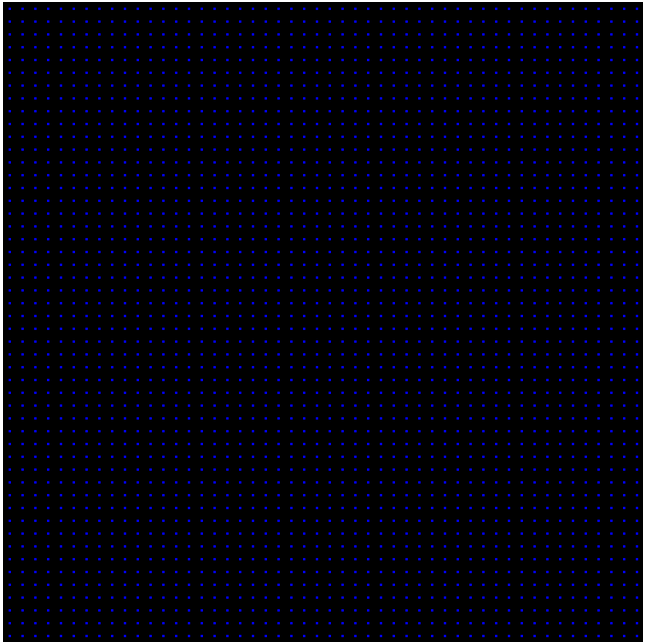Image with anchor centers
anchor_base = np.zeros((len(ratios) * len(scales), 4), dtype=np.float32)
print(anchor_base)
#Out:# array([[0., 0., 0., 0.],# [0., 0., 0., 0.],# [0., 0., 0., 0.],# [0., 0., 0., 0.],# [0., 0., 0., 0.],# [0., 0., 0., 0.],# [0., 0., 0., 0.],# [0., 0., 0., 0.],# [0., 0., 0., 0.]], dtype=float32)
for i in range(len(ratios)): for j in range(len(anchor_scales)): h = sub_sample * anchor_scales[j] * np.sqrt(ratios[i]) w = sub_sample * anchor_scales[j] * np.sqrt(1./ ratios[i])
 index = i * len(anchor_scales) + j
 anchor_base[index, 0] = ctr_y - h / 2. anchor_base[index, 1] = ctr_x - w / 2. anchor_base[index, 2] = ctr_y + h / 2. anchor_base[index, 3] = ctr_x + w / 2.
#Out:# array([[ -37.254833, -82.50967 , 53.254833, 98.50967 ],# [ -82.50967 , -173.01933 , 98.50967 , 189.01933 ],# [-173.01933 , -354.03867 , 189.01933 , 370.03867 ],# [ -56. , -56. , 72. , 72. ],# [-120. , -120. , 136. , 136. ],# [-248. , -248. , 264. , 264. ],# [ -82.50967 , -37.254833, 98.50967 , 53.254833],# [-173.01933 , -82.50967 , 189.01933 , 98.50967 ],# [-354.03867 , -173.01933 , 370.03867 , 189.01933 ]],# dtype=float32)

This is for one location, now we need to apply for all the anchor centers. since there are 50*50 anchor centers and each one has 9 anchors. in total we get 22500 anchors.

fe_size = 50anchors = np.zeros((fe_size * fe_size * 9), 4)
index = 0for c in ctr: ctr_y, ctr_x = c for i in range(len(ratios)): for j in range(len(anchor_scales)): h = sub_sample * anchor_scales[j] * np.sqrt(ratios[i]) w = sub_sample * anchor_scales[j] * np.sqrt(1./ ratios[i])
 anchors[index, 0] = ctr_y - h / 2. anchors[index, 1] = ctr_x - w / 2. anchors[index, 2] = ctr_y + h / 2. anchors[index, 3] = ctr_x + w / 2. index += 1
print(anchors.shape)#Out: [22500, 4]

A look at anchors at (400, 400) on image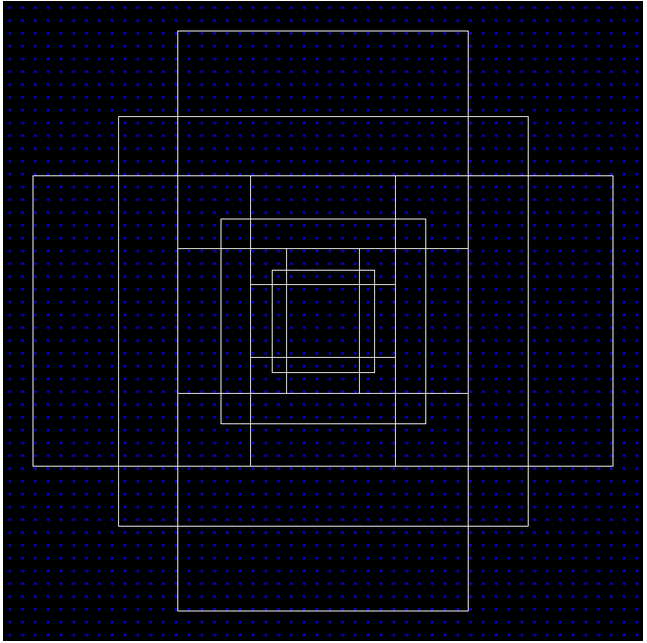Anchor boxes at (400, 400)
• So the RPN produces object proposals wrt to each anchor box along with their objectness score. An anchor box is assigned +ve if it has max_iou with the ground truth object or iou greater than 0.7. An anchor box is assigned negative if it has iou < 0.4. All the anchor boxes with iou [0.4, 0.7] are ignored. Anchor boxes which fall outside the image are also ignored.
• Again, since vast majority of them will have negative samples, we will use the same Fast R-CNN strategy to sample 128+ve samples and 128-ve samples (total 256) for a batch size of 2 for training.
• smooth L1 loss and cross entropy loss can be used for regression and classification. Regression outputs are offset with anchor box locations using the floowing formulas
t_{x} = (x - x_{a})/w_{a}t_{y} = (y - y_{a})/h_{a}t_{w} = log(w/ w_a)t_{h} = log(h/ h_a)

x, y , w, h are the groud truth box center co-ordinates, width and height. x_a, y_a, h_a and w_a and anchor boxes center cooridinates, width and height.

• Once RPN outputs are generated, we need to process them before sending to the RoI pooling layer (aka fast R-CNN network) . The Faster R_CNN says, RPN proposals highly overlap with each other. To reduced redundancy, we adopt non-maximum supression (NMS) on the proposal regions based on their cls scores. We fix the IoU threshold for NMS at 0.7, which leaves us about 2000 proposal regions per image. After an ablation study, the authors show that NMS does not harm the ultimate detection accuracy, but substantially reduces the number of proposals. After NMS, we use the top-N ranked proposal regions for detection. In the following we training Fast R-CNN using 2000 RPN proposals. During testing they evaluate only 300 proposals, they have tested this with various numbers and obtained this.
nms_thresh = 0.7n_train_pre_nms = 12000n_train_post_nms = 2000n_test_pre_nms = 6000n_test_post_nms = 300min_size = 16
• Once the ~2000 proposals are generated, these are transformed using the following formulas
x = (w_{a} * ctr_x_{p}) + ctr_x_{a}y = (h_{a} * ctr_x_{p}) + ctr_x_{a}h = np.exp(h_{p}) * h_{a}w = np.exp(w_{p}) * w_{a}
and later convert to y1, x1, y2, x2 format
• Now these 2000 proposals are similar to the proposals generated by selective search in Fast R-CNN. So the rest of the process is similar to Fast R-CNN.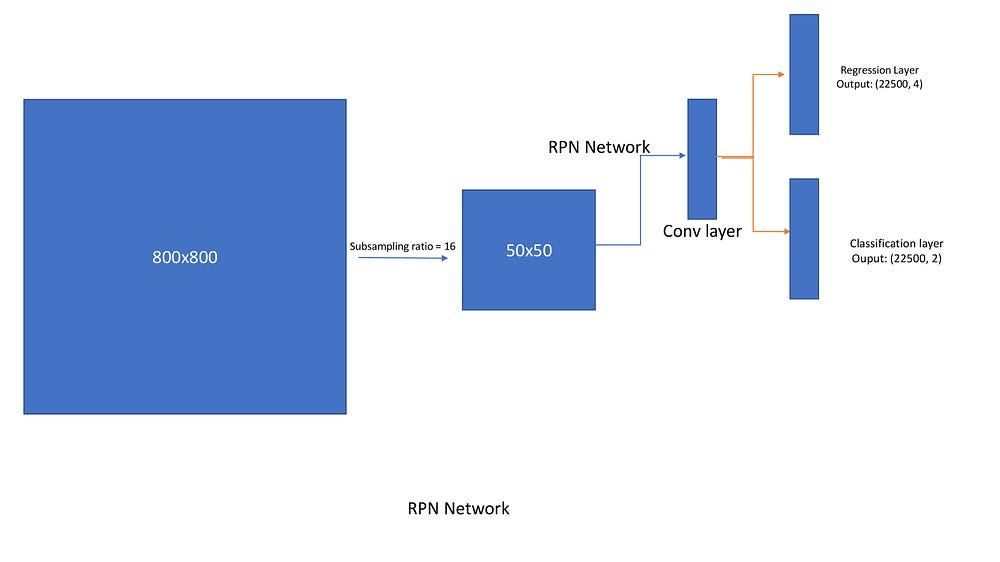Region Proposal network (RPN)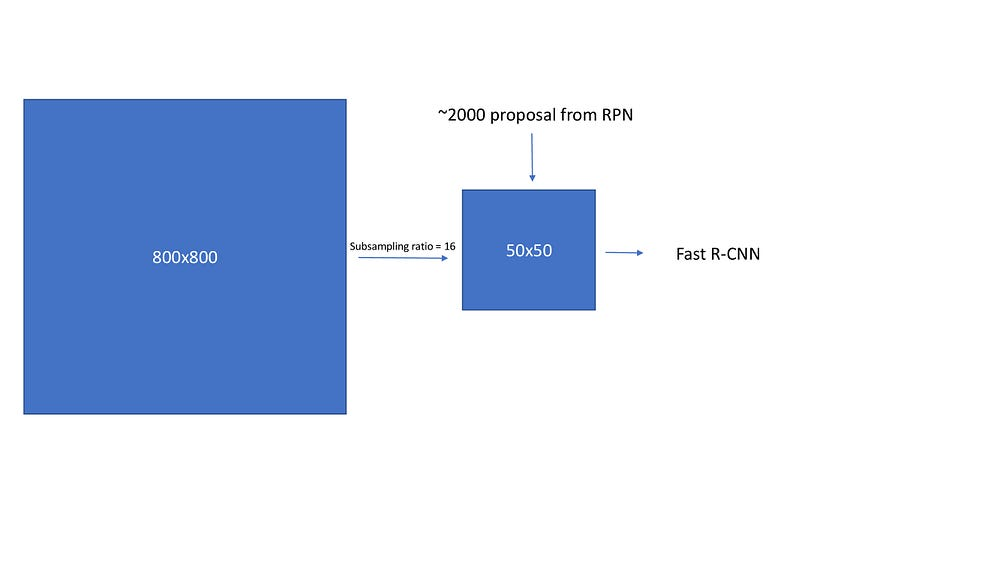Fast RCNN in Faster RCNN work flow

#### Results

• Inference at 5 FPS on a GPU
• Using VGG backend, with pretraining on ImageNet and COCO, On VOC Faster RCNN achieved 73.2% mAP.
• On COCO dataset, Faster R-CNN achieved 21.9 mAP (coco type).

### Feature Pyramid Networks

In this line, Researchers have observed two problems with the Faster R-CNN. First it is unable to detect small objects, second class imbalance is not focussed properly (Random sampling 256 samples and calculating loss is not a proper way). So the researches have introduced two new concepts

1. Feature Pyramid networks (FPN)
2. Focal Loss (For extreme class imbalance problem)

We will discuss these two techniques briefly here.

#### Feature Pyramid networks

If you look at the Faster RCNN, It is mostly unable to catch small objects in the image. This is largely addressed in COCO and ILSVRC challege using image pyramids by most of the winning teams. A simple image pyramid is given below, you scale image to different sizes and send it to the network, Once the detections are detected on each scale, all the predictions are combined using different method. Though this method worked, Inference is a costly process as each image should be computed at various scales independently.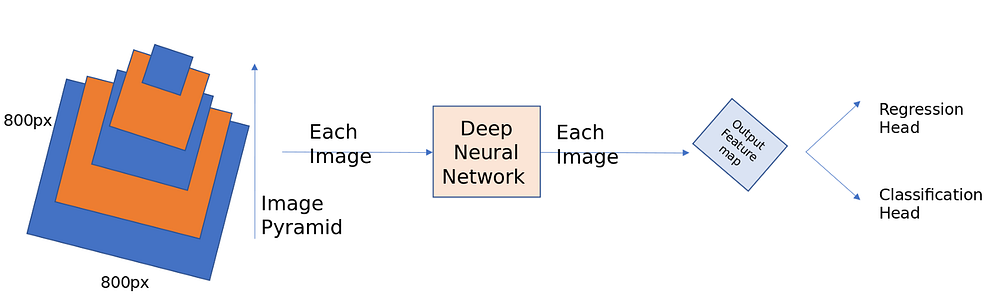Image Pyramids

A deep ConvNet computes a feature hierarchy layer by layer, and with sub-sampling layers the feature hierarchy has an inherent multi-scale, pyramidal shape. This in-network feature hierarchy produces feature maps of different spatial resolutions. First lets see how FPN works and later we will move into the intuition part.

• First take a standard resnet architecture(Ex ResNet50). In Faster R-CNN, discussed above, we have considered only feature maps of sub-sampling ratio 16 were taken to compute Region proposals and later pass the RPN outputs to Fast RCNN. Here it is done in the following way,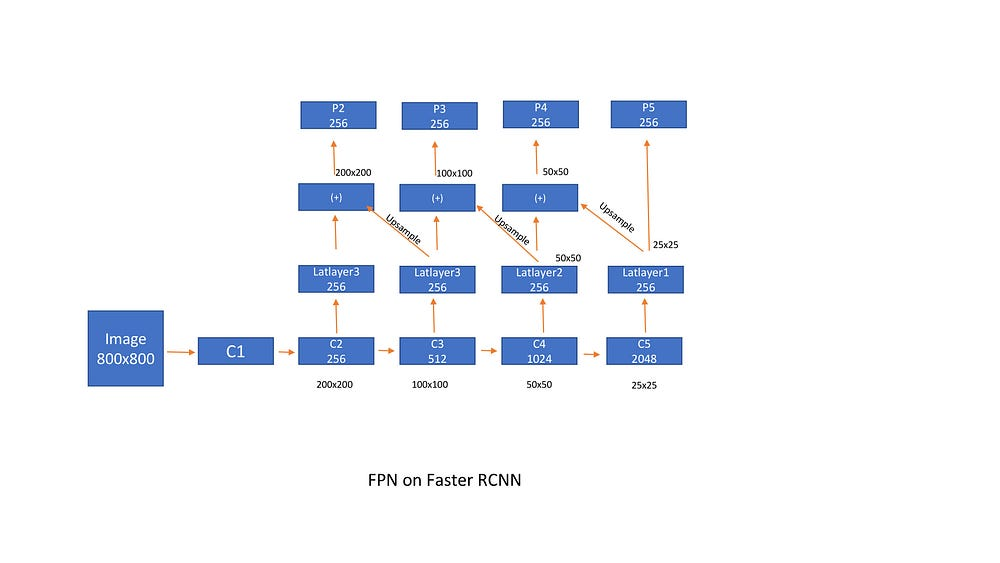FPN on Faster RCNN
• The problem with excessive sub sampling is it will lead to poor localization. If incase we want to use features from earlier layers (say subsample 8) the semantics of the object are not captured very clearly. So, we have to take the best of both the worlds. With this intuition feature pyramid networks or FPN is designed first on Faster RCNN. The latlayers reduces the channel dimension (number of feature maps), up-sampled and added to the previous layer outputs. Since up-sampling is done using bilinear interpolation, they have added another conv layer this output so that the aliasing effect of up-sampling is removed. We have ignored p1 because of the computational complexity (It generates (400*400*3 = 480k proposals for a single feature map p1).
• Anchor boxes are designed on each feature map separately. Since scale is taken care off by the FPN, At each location we take anchors of 3 aspect ratio [1:2, 2:1, 1:1]. In total we get 9 anchors at each location over the feature map scale.

#### FPN for RPN

• In Faster RCNN, a 3×3 conv layer slides over the feature map and extract features which further are evaluated by two sibling networks, one for regression (1×1 conv) and the other for classification (1×1 conv). Now while implementing FPN, we build the same network, but here we move the network on each and every feature map.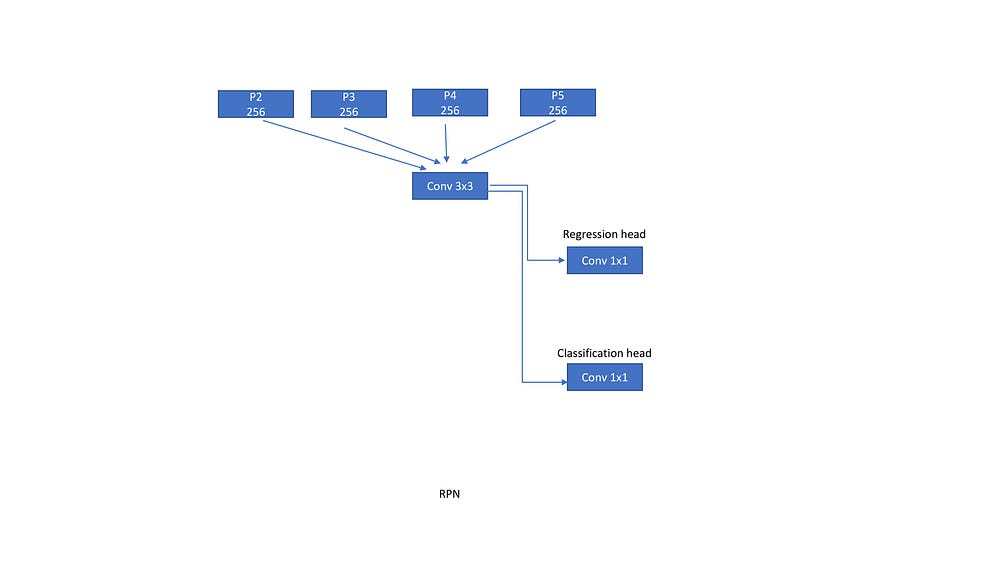Region Proposal Network

#### FPN for Fast R-CNN

• Similar to RPN, RoI pooling layer is attached to each feature map scale. A sub network predicts bounding boxes and classes for each scale separately. This sub network shares parameters across the scales same like in RPN.

#### Results:

• some ablation studies suggest that using FPN, Average precision has (AR^(1k)) improved by 8.0 points over a single scale RPN. It also boosted the performance of large margin by 12.9 points.
• lateral connections improved the AP by 10 points.
• The COCO AP stands at 33.9 and VOC AP@5 stands at 56.9. On small objects the coco style AP has improved from 9.6% to 17.8%.
• Using ResNet-101 as the backend, VOC AP stood at 59.1% and coco style mAP stood at 36.2%.
• Inference on each image takes 176 ms when using resnet101 as the backend.

### RetinaNet (Focal Loss and FPN combined)

Till now, All the techniques we have seen are two-stage, RPN generates object proposals and then Fast RCNN classifies and regress on top of those predicted object proposals. Now the question is can we build single stage detectors?

Two, when evaluating 1⁰⁴ to 1⁰⁵ anchor boxes in Faster RCNN (FPN) network , most of it doesn’t contain objects which leads to extreme class imbalance. To counter for class imbalance, we are sampling 256 proposal from each mini-batch (128+ve and 128-ve). However this is not a robost approach and the authors of this paper have proposed a loss function called Focal loss which tries to address class imbalance efficiently.

#### Single Stage Detector (FPN)

• As shown in the below diagram P3, P4, P5, P6, P7 are the outputs of the backend network. We are not using P1 and P2 because of computational reasons.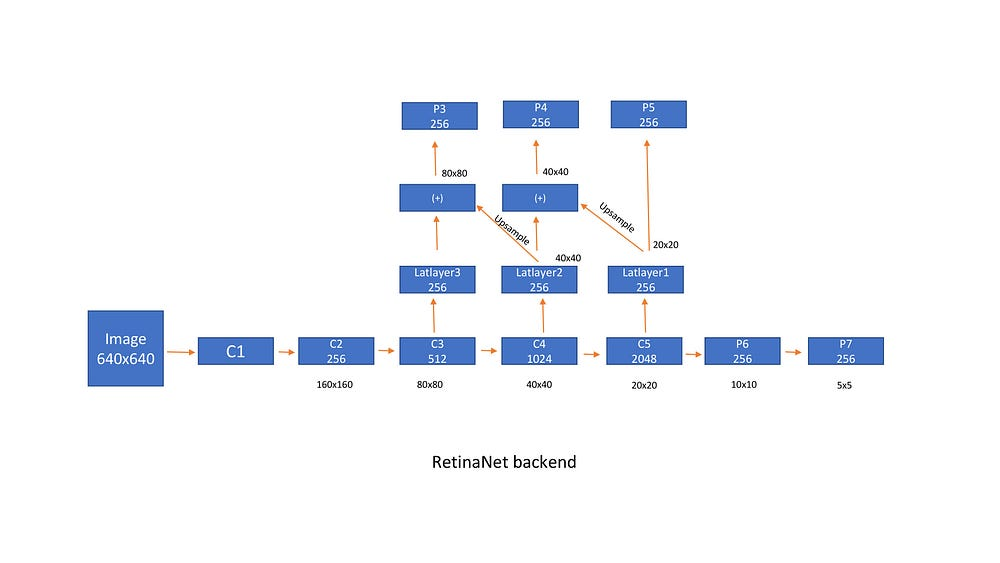RetinaNet backend
• Each output is then passed to a Regression layer and a classification layer, which are a bunch of conv layers (4) along with output layer. All the conv layers have 256 input channels and 256 output channels and applies a 3×3 sliding window. Relu is between all the layer. The regression layer output has a linear layer and the classification layer has a sigmoid (Number of classes as output) or softmax (num classes +1) activation function in the end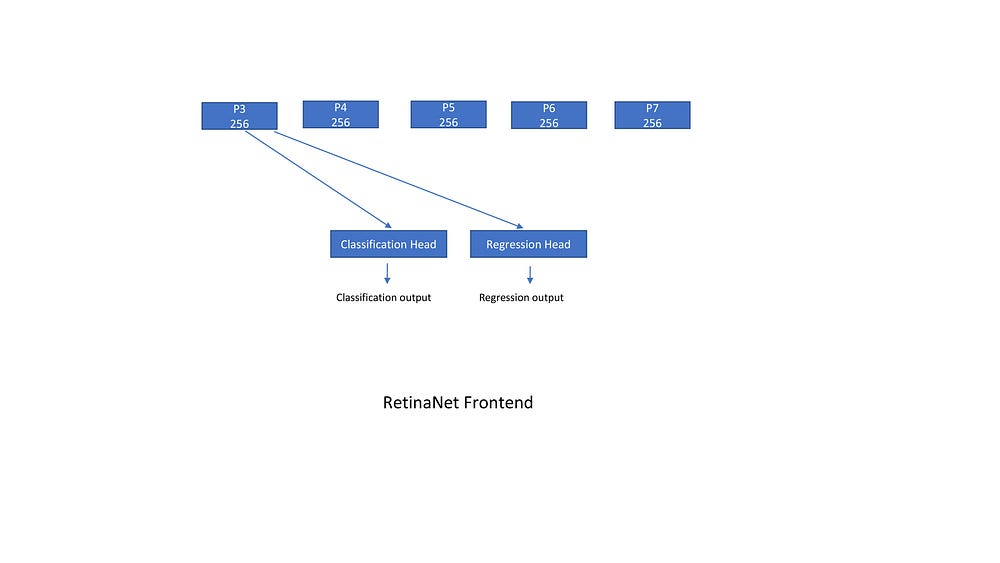RetinaNet FrontEnd
• The anchor boxes are designed in the same way as said in FPN section with some modifications. Strides are [8, 16, 32, 64, 128] for [p3, p4, p5, p6, p7] respectively. Though FPN takes care of scales, yet the paper reports better results using anchor boxes of different scales on each feature map. So we have 9 anchors at each location of feature map totaling to 45 anchors over all the scales. They used anchor boxes of sizes [²⁰, 2^(1/3), 2^(2/3)] and aspect ratios of [0.5, 1, 2]. sudo code is a follows
aspect_ratios = [0.5, 1, 2]kmin, kmax = 3, 7 #feature scalesscales_per_fe = 3 anchor_scale = 4 num_aspect_ratios = len(aspect_ratios)num=0
for lvl in range(k_min, k_max+1): stride = 2. ** lvl  for fe in range(scales_per_fe): fe_scale = 2**(fe/float(scales_per_fe)) for idx in range(num_aspect_ratios): anchor_sizes = (stride* fe_scale * anchor_scale,) anchor_aspect_ratios=(aspect_ratios[idx],) num+1=1 print(anchor_sizes, anchor_aspect_ratios)
#out
1 (32.0,) (0.5,)2 (32.0,) (1,)3 (32.0,) (2,)4 (40.317,) (0.5,)5 (40.317,) (1,)6 (40.317,) (2,)---43 (812.75,) (0.5,)44 (812.75,) (1,)45 (812.75,) (2,)
• encodings are the similar to Faster RCNN, If anchor box has iou > 0.5 with ground truth it is +ve, <0.4 it is -ve and remaining all are ignored. Each anchor is assigned to Atmost one anchor.
• For an Input size of 640×640, we get 8525 ((80*80) + (40*40) + (20*20) + (10*10) + (5*5)) locations. Since there are 9 anchors at each location we get a total of 76725 (8525×9) anchors.

#### Loss Function

Regression uses smooth L1 loss, which is less sensitive to outliers. This is the same loss used in all the frameworks till now.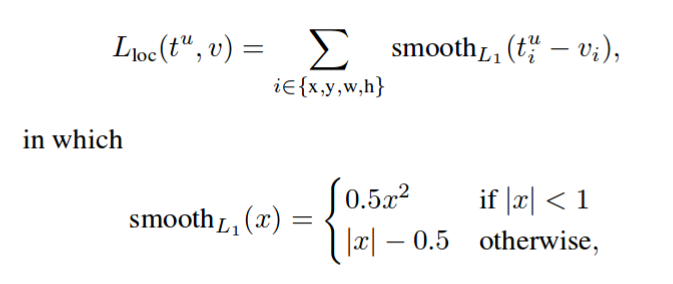smooth L1 loss

Classification loss is called focal loss, which is a reshaped version of cross entropy loss and this paper talks a lot about this.

• Focal loss is the reshaping of cross entropy loss such that it down-weights the loss assigned to well-classified examples. The novel focal loss focuses training on a sparse set of hard examples and prevents the vast number of easy negatives from overwhelming the detector during training.

Lets look at how this focal loss is designed. We will first look at binary cross entropy loss for single object classification

Cross entropy loss: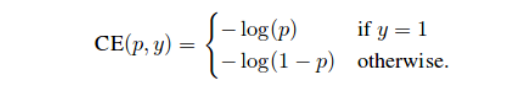cross entropy loss

Focal loss: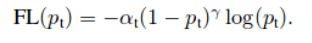Focal loss
• where p_{t} is p if y=1 and 1-p otherwise. Lets look at the focal loss equation clearly. Here alpha is called the balancing param and gamma is called the focusing param.
• balancing param is ususally the inverse of number of instances of specific class. It gives less weight to classes with more number of samples. Here to the negative proposals.
• Focusing param adds less weight to well classified examples and more weight hard/miss classified examples. Lets check how this is achieved using 4 scenarios. I have already explained this in my other blog and for the sake of completeness

#### Scenario-1: Easy correctly classified example

Say we have an easily classified foreground object with p=0.9. Now usual cross entropy loss for this example is

CE(foreground) = -log(0.9) = 0.1053

Now, consider easily classified background object with p=0.1. Now usual cross entropy loss for this example is again the same

CE(background) = -log(1–0.1) = 0.1053

Now, consider focal loss for both the cases above. We will use alpha=0.25 and gamma = 2

FL(foreground) = -1 x 0.25 x (1–0.9)**2 log(0.9) = 0.00026

FL(background) = -1 x 0.25 x (1–(1–0.1))**2 log(1–0.1) = 0.00026.

#### Scenario-2: misclassified example

Say we have an misclassified foreground object with p=0.1. Now usual cross entropy loss for this example is

CE(foreground) = -log(0.1) = 2.3025

Now, consider misclassified background object with p=0.9. Now usual cross entropy loss for this example is again the same

CE(background) = -log(1–0.9) = 2.3025

Now, consider focal loss for both the cases above. We will use alpha=0.25 and gamma = 2

FL(foreground) = -1 x 0.25 x (1–0.1)**2 log(0.1) = 0.4667

FL(background) = -1 x 0.25 x (1–(1–0.9))**2 log(1–0.9) = 0.4667

#### Scenario-3: Very easily classified example

Say we have an easily classified foreground object with p=0.99. Now usual cross entropy loss for this example is

CE(foreground) = -log(0.99) = 0.01

Now, consider easily classified background object with p=0.01. Now usual cross entropy loss for this example is again the same

CE(background) = -log(1–0.01) = 0.1053

Now, consider focal loss for both the cases above. We will use alpha=0.25 and gamma = 2

FL(foreground) = -1 x 0.25 x (1–0.99)**2 log(0.99) = 2.5*10^(-7)

FL(background) = -1 x 0.25 x (1–(1–0.01))**2 log(1–0.01) = 2.5*10^(-7)

#### Conclusion:

scenario-1: 0.1/0.00026 = 384 times smaller number

scenario-2: 2.3/0.4667 = 5 times smaller number

scenario-3: 0.01/0.00000025 = 40,000 times smaller number.

These three scenarios clearly show that Focal loss add very less weight to well classified examples and large weight to miss-classified or hard classified examples.

#### Inference on RetinaNet

This is the basic intuition behind designing Focal loss. The authors have tested different values of alpha and gamma and final settled with the above mentioned values.

• 1000 proposals from each feature scale were obtained after thresholding detector confidence at 0.05. The top predictions from all levels are merged and non-maximum suppression with a threshold of 0.5 is applied to yield the final detections.

Note: Please be careful while initializing the network with random weights. The authors have suggested to use (-1) x math.log((1–0.01)/0.01) for the last layer bias. If not done, we get a huge loss value in the first iteration and the gradients overflow, which ultimately leads to NaNs.

#### Results

• On COCO dataset, Retinanet achieved an coco style mAP of 40.8 and VOC style mAP at 61.1 mAP. coco style AP at small objects is 24.1, medium objects is 44.2 and Large objects is 51.2.
• Using 600×600 input sizes and resnet101 as backend, inference takes 122ms on each image.

### Yolo (You look only once)

Apart from this research and evolution of these frameworks. There are other researches who have proposed similar approaches to improve the speed and accuracy. One is SSD and the other is Yolo. we will look into the Yolo framework first. Yolo has evolved over a period of time and has published 3 papers till now. Though most of the ideas are similar to the ones we have discussed above, it is good to look at these approaches as it largely addresses the speed problem.

• Yolo has three papers written till to date, Yolo1, yolo2, Yolo3 which showed improvements in terms of accuracy.
• Yolo1 is independently published and to my knowledge is the first paper which talks about single stage detection. RetinaNet takes ideas from Yolo and SSD.

#### Grid cells

• First we divide the image into a grid of cells. Say it is 7×7. Now ground truth objects which have their object centers in their respective grid cells turns out to be +ve cells.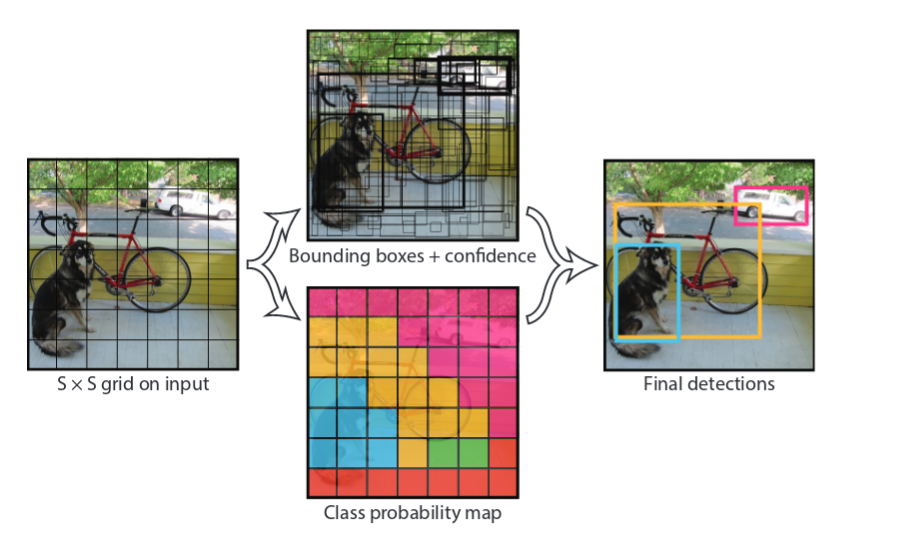Yolo grid cell
• Each cell predicts 2 bounding boxes and each bounding box consists of 5 predictions :x,y,w,h, and confidence. The (x,y) coordinates represent the center of the box relative to the bounds of the grid cell. The width and height are predicted relative to the whole image. Finally the confidence prediction represents the IOU between the predicted box and any ground truth box. So for VOC with 20 classes the output would be ((4+1)x2+20) = 30. If the grid size is 7×7, we will have 7x7x30 outputs.
def normal_to_yolo_format(bbox, anchor, img_size, grid_size): y, x, h, w = bbox #bounding box y1, x1, y2, x2 = anchor # supporting cell
 size_h = img_size/ grid_size # height of the cell size_w = img_size/grid_size # width of the cell new_x = (x - x1)/size_w # center_x wrt to cell new_y = (y - y1)/size_h #center_y wrt to cell new_h = h/img_size # height of the object normalized with the heigth of the image new_w = w/img_size # width of the object normalized with the width of the image return [new_y, new_x, new_h, new_w]

#### Encoding bounding boxes

For each cell, For each ground_truth_bbox: check if the cell contains bbox center or not, if yes: encode the box with respective values of bbox, label and binary encoding of object present or not[1 in this case].

#### Problem with encodings:

What will happen if the grid cell contains two object centers ? You either can take randomly choose one of the object or increase the grid size to a much bigger number like 16×16. This is largely addressed in Yolov2 and we will have look at der.

#### Network

Yolo uses a different network called DarkNet, which is home brewed by the author unlike other frameworks which takes standard well performed ImageNet architectures.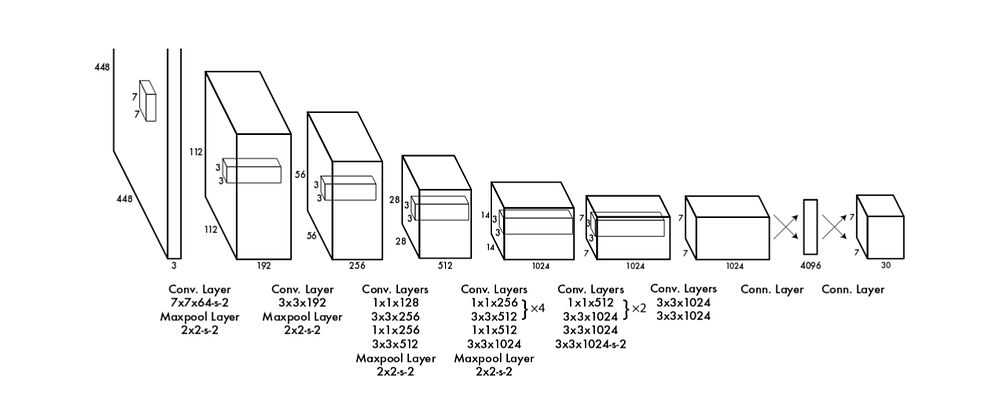Yolo network

#### Loss functions.

Yolo contains three loss functions.

1. Coordinates error
2. Objectness score
3. Classification error.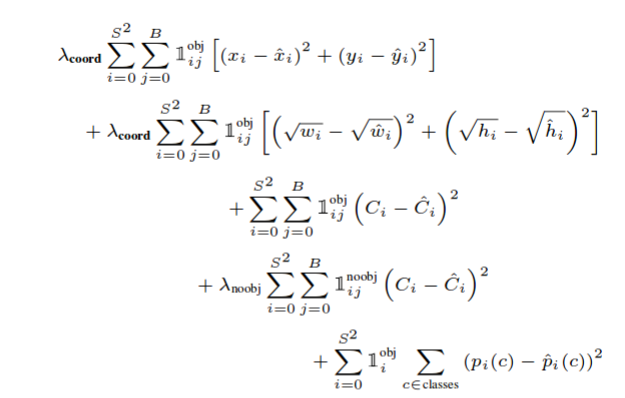Yolo loss

Coordinate error and classification error are only evaluated if the objectness score is >0.5, which is represented with 1^obj . The loss is calculated individually for each grid cells and everything is summed later. w and h have square roots to address the following issue

• A small object predicting wrong should be given more weight than a large object predicting it wrong.

\lambda_coord and \lambda_noobj are 5 and 0.5 respectively.

In my experience getting this loss function correctly for my own datasets became a nightmare. may be these loss functions are tuned to work for this dataset and might work for datasets with similar distribution. It might heavily fail when you are working for other datasets. I will do another blog post on issues I faced while building object detection pipelines from scratch.

#### Training

• The DarkNet is first Pre-trained on Imagenet for classification. Top-5 accuracy stood at 88%. Since detection requires fine grained information they have used 448×448 images rather than 224×224. They found that the errors are high in the initial epochs and thought that it might have been because of change in image sizes. so they have again trained the network on imagenet using 448×448 for the first few epochs and later moved on to voc datasets.
• The network is later trained on VOC-2007 and 2012 dataset for 135 epochs. Throughout training they used a batch_size of 64, momentum of 0.9 and a decay of 0.0005. The learning rate schedule is as follows, For the first epochs they slowly raised the learning rate from 10^-3 to 10^-2. Continued training with 10^-2 for 75 epochs, then 10^-3 for 30 epochs and finally 10^-4 for 30 epochs.
• To avoid over-fitting they used dropout and extensive data augmentation. Here in our case, we have used batch normalization also.
• For data augmentation they have used random scaling and translations of up to 20% of the original image size. They also randomly adjust the exposure and saturation of the image by up to a factor of 1.5 in the HSV color space.

#### Results

• Yolo is extremely fast. It works at 45 frames per second
• YOLO using DarkNet achieves an mAP of 63.4 at 45 frames per second. mAP is far less compared to Faster R-CNN using VGG-16 (73.2) but the speed of Faster R-CNN is at 7 frames per second. The Fast R-CNN framework achieves an mAP of 71.8% at 0.5 FPS (frames per second).
• Yolo imposes strong spatial constraints on bounding box predictions since each grid cell only predicts two boxes and can only have one class. This spatial constraint limits the number of nearby objects that our model can predict. For example, It will be very hard for Yolo to predict a flock of animals (birds).
• Since the model predicts bounding boxes from data, it struggles to generalize to objects in new or unusual aspect ratios and configurations.
• Yolo is better at making less localization errors as it looks at the entire image to predict objects on individual cells. So Yolo captures and considers global features of the entire image for predicting for individual cells, which is not the case with Faster R-CNN. A survey on the errors found that Fast R-CNN almost make 3x background errors compared to Yolo. So for every bounding box the Fast R-CNN predicts, it is again evaluated by the Yolo. This improved the mAP of Fast R-CNN by 3.2% to 75%.

#### Yolov2

I am not going to discuss in-depth of Yolov2 as most of the ideas are copied from other papers or are already discussed already. I will just highlight the modifications they bought to Yolo in this paper

• At 67 FPS, YOLOv2 gets 76.8 mAP on VOC 2007. At 40 FPS, YOLOv2 gets 78.6 mAP, outperforming state-of-the-art methods like Faster R-CNN with ResNet and SSD while still running significantly faster.
• Yolo uses anchor boxes at each grid cell. this will allow us to encode multiple boxes in the same grid cells.
• Scales and aspect ratios for the bounding boxes for anchor boxes are set using dataset. (I will write a separate write up on this as it can be used in all the other frameworks too). Setting better priors and understanding the dataset is of primary importance and is first addressed in Yolov2 paper only.
• Grid size increased from 7×7 to 13×13. This also should come from the dataset. If you have extremely small objects we can increase this to 32×32 or 50×50 according to the need. One of the prior checks we need to make when considering grid size is, No anchor boxes should more than one ground-truth box.
• To the network, Batch normalization was introduced. Similar to Network In Network, they used global average pooling and 1×1 convolutions.
• Encoding of x, y, w, h has changed in the following way. This were the same equations used in Yolov3. This is done because directly predicting object locations on to image is leading to model instability and again they are not constrained also.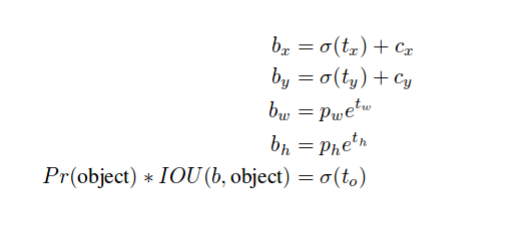Yolov2 bounding box encoding
• Multi-scale training. nstead of fixing the input image size we change the net-work every few iterations. Every 10 batches our network randomly chooses a new image dimension size. Since our model down samples by a factor of 32, we pull from the following multiples of 32: {320, 352, …, 608}. Thus the smallest option is 320×320 and the largest is 608×608. We resize the network to that dimension and continue training.

#### Yolov3

Yolov3 paper is a fun read and I will highlight the most important things here.

• Yolov3 achieves 33.4 IOU@0.5:0.95 mAP and 57.9 IOU@0.5 mAP. The author takes a dig on why we switched our metrics and supports IOU@0.5(VOC style). Using VOC style mAP, Yolov3 is very robust
• Yolov3 replaces darknet19 with darknet53 and introduces residual blocks. It also introduces FPN. The networks look as shown below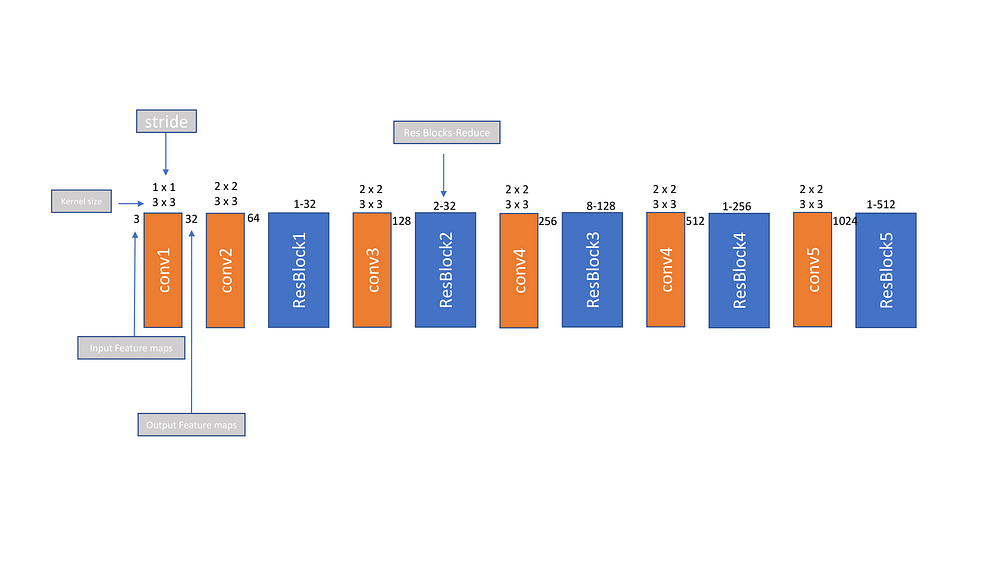Darknet53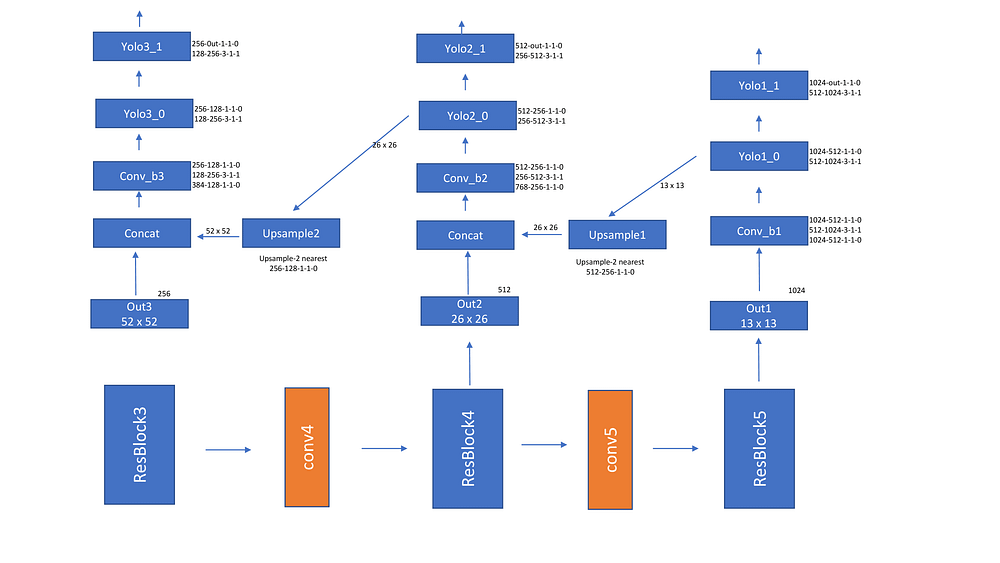Yolov3 Network
• The loss function is similar to Yolov2 and Yolov1 except that it uses binary cross entropy. In my experiments this didn’t work and I have used MSE loss divided the number of positive bounding boxes.
• Encoding is done in the following way. A gt box is attached to the anchor which has max_iou. Only anchors of the cell which contain the gt_box center are considered for the anchors.
• Since Yolov3 uses 3 scales, 52×52, 26×26 and 13×13. The bounding boxes are encoded individually on each scale. loss is calculated on each layer individually and later summed.

### SSD — Single shot detector

• This is the last framework we will be discussing in this blog post and I believe almost all the ideas are already discussed already. So readers who are interested to have full depth knowledge can go and read the paper and I believe after the knowledge you have acquired from this blog post, it should be a very simple read.
• Released after YOLO and Faster RCNN, this paper achieves 74.3 mAP at 59fps for 300×300 input size images, We call this network SSD300. Similarly SSD512 achieves 76.9% mAP surpassing Faster R-CNN results.

#### Network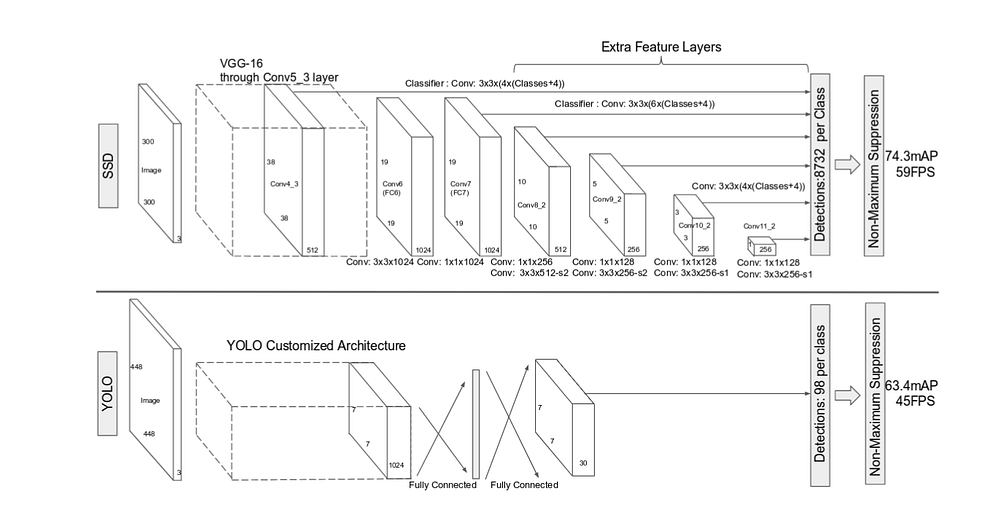SSD

The paper itself compares it network with YOLO. It uses a VGG backend and extract features from multiple scales. Note that, it doesn’t use any lateral connections like Yolov3 are FPN.

#### Anchor box design

• Since feature maps from different layers already captures scales, SSD uses only one scale per layer, however the scales are decided using a formula sk = smin + (smax — smin)/(m-1) * (k-1), where smin is 0.2 and smax is 0.9.
• Aspect ratios of [1, 2, 3, 1/2, 1/3] are used. w and h for each box are decided using sk * a^(1/2) and sk/a^(1/2) . They have also added an anchor box of (sk * sk+1)^(1/2), totaling to 6 anchor boxes per locations
• As shown in the diagram conv4_3 (38×38), conv7 (fc7)(19×19), conv8_2(10×10), conv9_2(5×5), conv10_2(3×3), and conv11_2(1×1). The authors have suggested to use [4, 6, 6, 6, 4, 4] anchors at each features scale. Total anchors = (38x38x4) + (19x19x6) + (10x10x6) + (5x5x6) + (3x3x4) + (1x1x4) = (8732 anchors)

#### Encoding

• SSD uses Jaccard overlap and all the anchor boxes which have overlap >0.5 are considered +ve.

#### Loss functions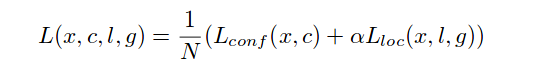Loss SSD

For confindence, cross-entropy loss is used. For loc, smooth L1 loss is used. Unlike Faster R-CNN, to address class imbalance they didn’t just randomly sample +ve and -ve boxes. They have ordered the objectness score in decreasing order for the negative samples and took the top boxes. In this way they got a 3:1 -ve to +ve samples ratio which lead to faster convergence.

This is it. We have discussed almost all the state of the art papers in object detection. There are so many other papers to improve the mAP (Ex GroupNorm instead of BatchNorm), train faster (Ex Use SGDR) to discuss. But for now we have to end this here.

#### References

Thank you.

If you have any suggestions please write to vanapalli.prakash@fractalanalytics.com or just mention in the comments section.